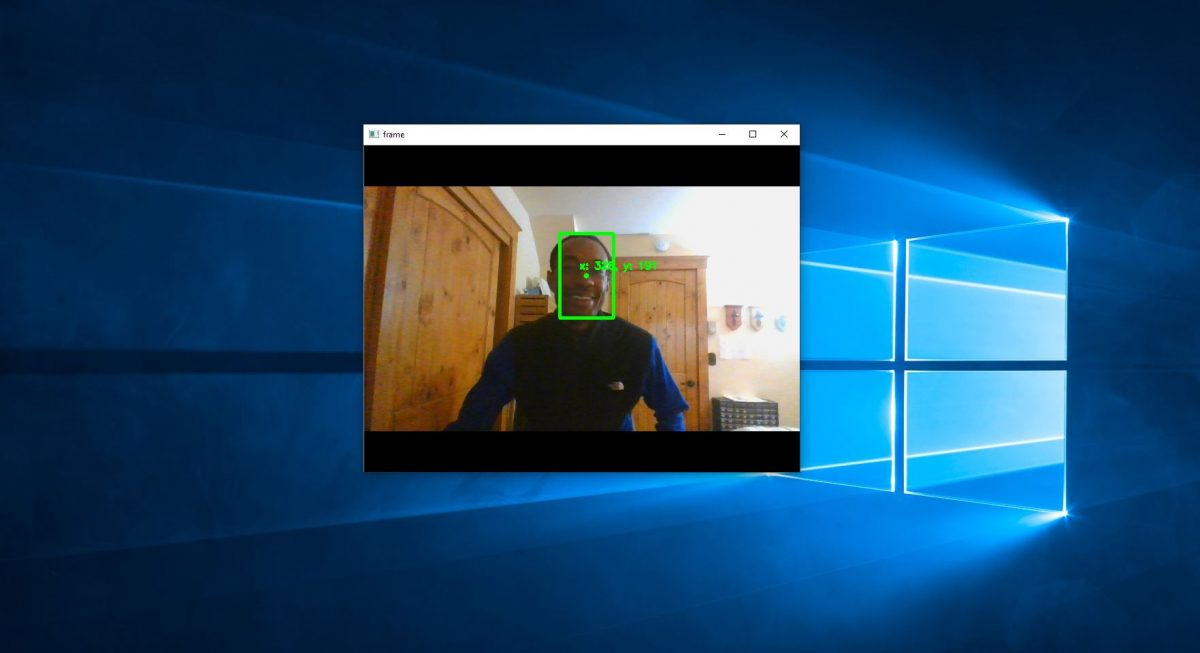# Real-Time Object Tracking Using OpenCV and a WebcamIn this tutorial, we will create a program to track a moving object in real-time using the built-in webcam of a laptop computer. We will use Python and the OpenCV computer vision library for the code.

A real-world application of this is in robotics. Imagine you have a robot arm that needs to continuously pick up moving items from a conveyor belt inside a warehouse. In order for the robot to pick up an object it needs to know the exact coordinates of the object. The program we will create below will give you the basic building block to do just that. It will locate the coordinates of the center of the moving object (often called the “centroid“) in real-time using an ordinary webcam.

Let’s get started!

# Prerequisites

• Python 3.7 or higher

# Requirements

Using real-time streaming video from your built-in webcam, create a program that:

• Draws a bounding box around a moving object
• Calculates the coordinates of the centroid of the object
• Tracks the centroid of the object

# Directions

Open up your favorite IDE or code editor.

Make sure you have the OpenCV and Numpy libraries installed. There are a number of ways to install both libraries. The most common way is to use pip, which is the standard package manager for Python.

``pip install opencv-python``
``pip install numpy``

Copy and paste the code below. This is all you need to run the program.

I put detailed comments inside the code so that you know what is going on. The technique used here is background subtraction, one of the most common ways to detect moving objects in a video stream:

```#!/usr/bin/env python

'''
Welcome to the Object Tracking Program!

Using real-time streaming video from your built-in webcam, this program:
- Creates a bounding box around a moving object
- Calculates the coordinates of the centroid of the object
- Tracks the centroid of the object

Author:
'''

from __future__ import print_function # Python 2/3 compatibility
import cv2 # Import the OpenCV library
import numpy as np # Import Numpy library

# Project: Object Tracking
# Date created: 06/13/2020
# Python version: 3.7

def main():
"""
Main method of the program.
"""

# Create a VideoCapture object
cap = cv2.VideoCapture(0)

# Create the background subtractor object
# Use the last 700 video frames to build the background
back_sub = cv2.createBackgroundSubtractorMOG2(history=700,

# Create kernel for morphological operation
# You can tweak the dimensions of the kernel
# e.g. instead of 20,20 you can try 30,30.
kernel = np.ones((20,20),np.uint8)

while(True):

# Capture frame-by-frame
# This method returns True/False as well
# as the video frame.

# Use every frame to calculate the foreground mask and update
# the background

# Close dark gaps in foreground object using closing

# Remove salt and pepper noise with a median filter

# Threshold the image to make it either black or white

# Find the index of the largest contour and draw bounding box
areas = [cv2.contourArea(c) for c in contours]

# If there are no countours
if len(areas) < 1:

# Display the resulting frame
cv2.imshow('frame',frame)

# If "q" is pressed on the keyboard,
# exit this loop
if cv2.waitKey(1) & 0xFF == ord('q'):
break

# Go to the top of the while loop
continue

else:
# Find the largest moving object in the image
max_index = np.argmax(areas)

# Draw the bounding box
cnt = contours[max_index]
x,y,w,h = cv2.boundingRect(cnt)
cv2.rectangle(frame,(x,y),(x+w,y+h),(0,255,0),3)

# Draw circle in the center of the bounding box
x2 = x + int(w/2)
y2 = y + int(h/2)
cv2.circle(frame,(x2,y2),4,(0,255,0),-1)

# Print the centroid coordinates (we'll use the center of the
# bounding box) on the image
text = "x: " + str(x2) + ", y: " + str(y2)
cv2.putText(frame, text, (x2 - 10, y2 - 10),
cv2.FONT_HERSHEY_SIMPLEX, 0.5, (0, 255, 0), 2)

# Display the resulting frame
cv2.imshow('frame',frame)

# If "q" is pressed on the keyboard,
# exit this loop
if cv2.waitKey(1) & 0xFF == ord('q'):
break

# Close down the video stream
cap.release()
cv2.destroyAllWindows()

if __name__ == '__main__':
print(__doc__)
main()
```